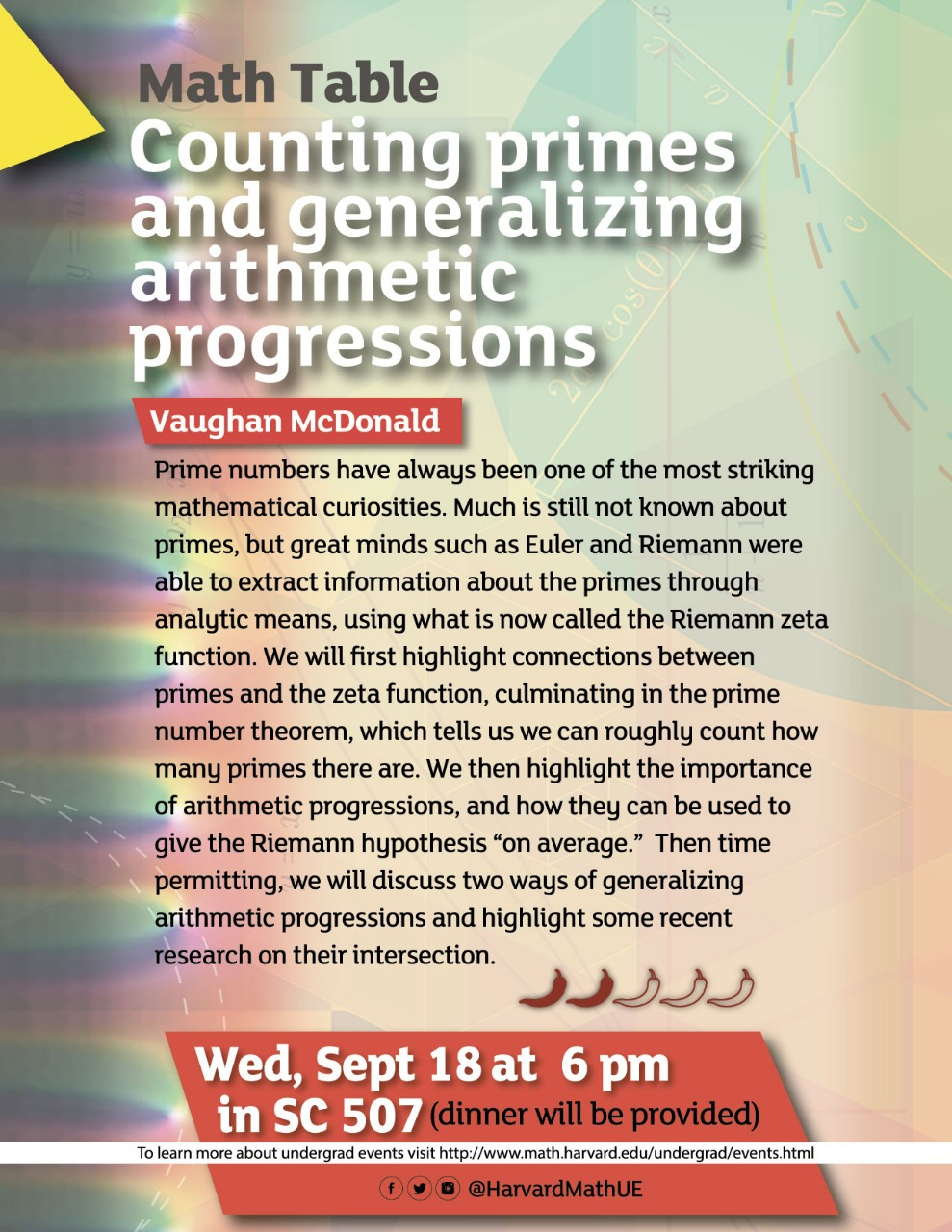Department of Mathematics FAS Harvard University One Oxford Street Cambridge MA 02138 USA Tel: (617) 495-2171 Fax: (617) 495-5132

### Math table September 18, 2019The first official Math Table talk will be on September 18th at 6pm in SC 507. Dinner is provided: Speaker: Vaughan McDonald Title: Counting primes and generalizing arithmetic progressions Abstract: Prime numbers have always been one of the most striking mathematical curiosities. Much is still not known about primes, but great minds such as Euler and Riemann were able to extract information about the primes through analytic means, using what is now called the Riemann zeta function. We will first highlight connections between primes and the zeta function, culminating in the prime number theorem, which tells us we can roughly count how many primes there are. We then highlight the importance of arithmetic progressions, and how they can be used to give the Riemann hypothesis "on average." Then time permitting, we will discuss two ways of generalizing arithmetic progressions and highlight some recent research on their intersection.